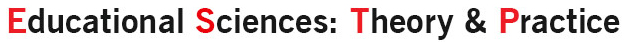Educational Sciences: Theory & Practice

## ISSN: 2630-5984## Analysis of Sixth Grade Students’ Think-Aloud Processes While Solving a Non-routine Mathematical Problem

##### Zeynep Çiğdem Özcan
Department of Mathematics and Science Education, Maltepe University, Istanbul Turkey
##### Yeşim İmamoğlu
Department of Mathematics and Science Education, Boğaziçi University, Istanbul Turkey.
##### Vildan Katmer Bayraklı
Department of Mathematics and Science Education, Maltepe University, Istanbul Turkey.

## Abstract

Problem solving is highlighted in many mathematics curricula and has recently become one of the most investigated topics in the field of mathematics education. Extensive studies report that developing students’ problem solving skills enhances their understanding of mathematics. Therefore, there is a focus on investigating problem solving processes of students. These processes are analyzed using various measurement techniques where data are collected in written or verbal forms. One of the techniques using verbal data is the “think-aloud method.” This study investigates sixth grade students’ think-aloud processes while solving a mathematical problem verbally. The study group consists of 24 sixth grade students selected according to the results of a problem solving test developed by the researchers. The think-aloud process of each student was videotaped and transcribed. Collected data were coded and categorized. Frequencies of categories were determined and compared according to students’ performance at solving mathematical problems. Results indicate that students have difficulty in expressing their thoughts during problem solving. Most students attempted operations with the numbers given, spending almost no time on understanding the problem. Some of the students who did the necessary computations correctly could not reach the solution because they could not interpret the result of the operation.

##### Keywords
Mathematical problem solving, Verbal data, Think-aloud method, Mathematical thinking process, Problem solving performances.